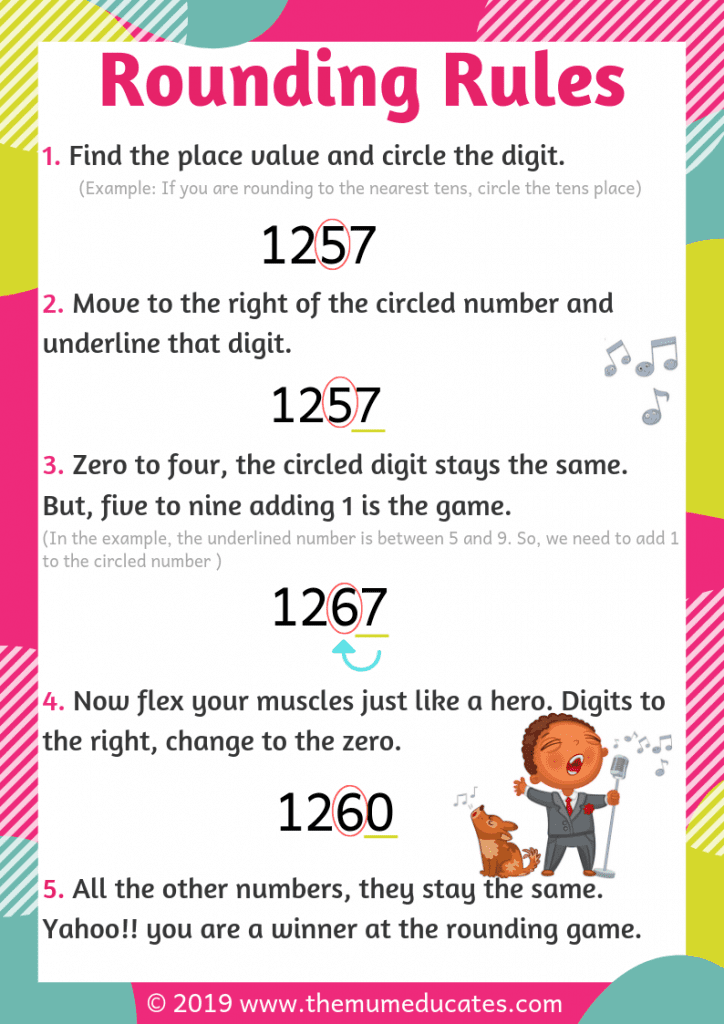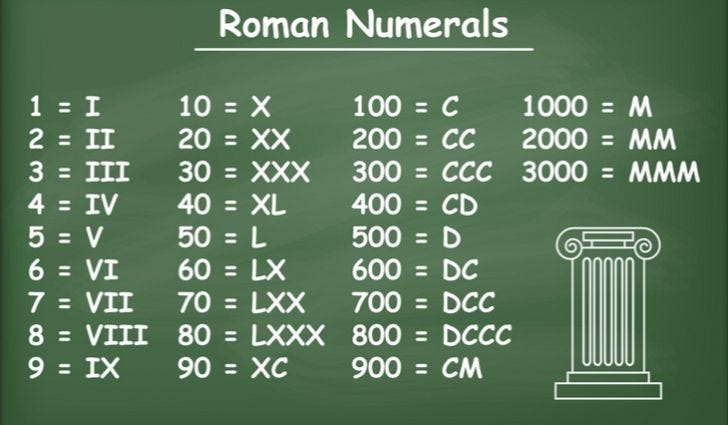# Place Value

TH  H  T  O  .  t  h

In Year 4, children are expected to work mathematically with four digit numbers before extending their knowledge to negative numbers and decimal places.

Using place value knowledge, they should be able to partition 4-digit numbers, place them on a number line, find 1000 more or less, and order and compare numbers.Rounding to the nearest 10, 100 and 1000

Here's the general rule for rounding:

1. If the number you are rounding is followed by 5, 6, 7, 8, or 9, round the number up. Example: 38 rounded to the nearest ten is 40. ...
2. If the number you are rounding is followed by 0, 1, 2, 3, or 4, round the number down. Example: 33 rounded to the nearest ten is 30.Negative NumbersRoman NumeralsTop